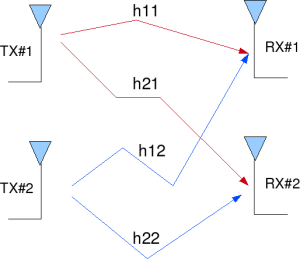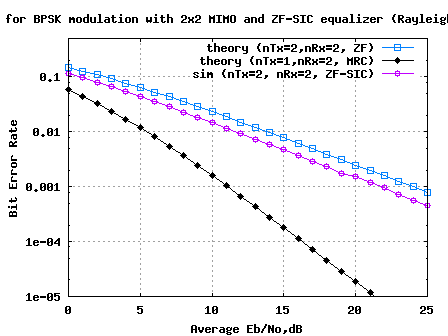# MIMO with Zero Forcing Successive Interference Cancellation equalizer

The post on MIMO with Zero Forcing equalizer discussed a probable way of equalizing a 2×2 MIMO channel. The simulated results with the 2×2 MIMO system with zero forcing equalizer showed matching results as obtained in for a 1×1 system for BPSK modulation in Rayleigh channel. In this post, we will try to improve the bit error rate performance by trying out Successive Interference Cancellation (SIC). We will assume that the channel is a flat fading Rayleigh multipath channel and the modulation is BPSK.

The background material on the MIMO channel has been described in the post on Zero Forcing equalizer. The text is repeated again for easy readability.

## 2×2 MIMO channel

In a 2×2 MIMO channel, probable usage of the available 2 transmit antennas can be as follows:

1. Consider that we have a transmission sequence, for example

2. In normal transmission, we will be sending in the first time slot, in the second time slot, and so on.

3. However, as we now have 2 transmit antennas, we may group the symbols into groups of two. In the first time slot, send and from the first and second antenna. In second time slot, send and from the first and second antenna, send and in the third time slot and so on.

4. Notice that as we are grouping two symbols and sending them in one time slot, we need only time slots to complete the transmission – data rate is doubled !5. This forms the simple explanation of a probable MIMO transmission scheme with 2 transmit antennas and 2 receive antennas.Figure: 2 Transmit 2 Receive (2×2) MIMO channel

## Other Assumptions

1. The channel is flat fading – In simple terms, it means that the multipath channel has only one tap. So, the convolution operation reduces to a simple multiplication. For a more rigorous discussion on flat fading and frequency selective fading, may I urge you to review Chapter 15.3 Signal Time-Spreading from [DIGITAL COMMUNICATIONS: SKLAR]2. The channel experience by each transmit antenna is independent from the channel experienced by other transmit antennas.

3. For the transmit antenna to receive antenna, each transmitted symbol gets multiplied by a randomly varying complex number . As the channel under consideration is a Rayleigh channel, the real and imaginary parts of are Gaussian distributed having mean and variance .

4. The channel experienced between each transmit to the receive antenna is independent and randomly varying in time.

5. On the receive antenna, the noise has the Gaussian probability density function with

with and .

7. The channel is known at the receiver.

## Zero forcing equalizer for 2×2 MIMO channel

Let us now try to understand the math for extracting the two symbols which interfered with each other. In the first time slot, the received signal on the first receive antenna is,

.

.

where

, are the received symbol on the first and second antenna respectively,

is the channel from transmit antenna to receive antenna,

is the channel from transmit antenna to receive antenna,

is the channel from transmit antenna to receive antenna,

is the channel from transmit antenna to receive antenna,

, are the transmitted symbols and

is the noise on receive antennas.

For convenience, the above equation can be represented in matrix notation as follows:

.

Equivalently,

To solve for , The Zero Forcing (ZF) linear detector for meeting this constraint . is given by,

.

To do the Successive Interference Cancellation (SIC), the receiver needs to perform the following:

## Zero Forcing with Successive Interference Cancellation (ZF-SIC)

Using the Zero Forcing (ZF) equalization approach described above, the receiver can obtain an estimate of the two transmitted symbols , , i.e.

.

Take one of the estimated symbols (for example ) and subtract its effect from the received vector and , i.e.

.

Expressing in matrix notation,

,

The above equation is same as equation obtained for receive diversity case. Optimal way of combining the information from multiple copies of the received symbols in receive diversity case is to apply Maximal Ratio Combining (MRC).

The equalized symbol is,

.

This forms the simple explanation for Zero Forcing Equalizer with Successive Interference Cancellation (ZF-SIC) approach.

## Simulation Model

The Matlab/Octave script performs the following

(a) Generate random binary sequence of +1’s and -1’s.

(b) Group them into pair of two symbols and send two symbols in one time slot

(c) Multiply the symbols with the channel and then add white Gaussian noise.

(d) Equalize the received symbols with Zero Forcing criterion

(e) Take the symbol from the second spatial dimension, subtract from the received symbol

(f) Perform Maximal Ratio Combining for equalizing the new received symbol

(g) Perform hard decision decoding and count the bit errors

(h) Repeat for multiple values of and plot the simulation and theoretical results.Figure: BER plot for BPSK in 2×2 MIMO channel with Zero Forcing Successive Interference Cancellation equalization

## Observations

Compared to Zero Forcing equalization alone case, addition of successive interference cancellation results in around 2.2dB of improvement for BER of .

The improvement is brought in because decoding of the information from the first spatial dimension () has a lower error probability that the symbol transmitted from the second dimension. However, the assumption is that is decoded correctly may not be true in general. We can discuss alternate approaches in future posts.## References

[DIG-COMM-BARRY-LEE-MESSERSCHMITT] Digital Communication: Third Edition, by John R. Barry, Edward A. Lee, David G. Messerschmitt[WIRELESS-TSE, VISWANATH]Fundamentals of Wireless Communication, David Tse, Pramod Viswanath%%%%%%%%%%%%%%%%%%%%%%%%%%%%%%%%%%%%%%%%%%%%%%%%%%%%%%%%%%%%
% The file may not be re-distributed without explicit authorization
% from Krishna Pillai.
% Checked for proper operation with Octave Version 3.0.0
% Author        : Krishna Pillai
% Email         : krishna@dsplog.com
% Version       : 1.0
% Date          : 09 Novemeber 2008
% %%%%%%%%%%%%%%%%%%%%%%%%%%%%%%%%%%%%%%%%%%%%%%%%%%%%%%%%%

% Script for computing the BER for BPSK modulation in a
% Rayleigh fading channel with 2 Tx, 2Rx MIMO channel
% Zero Forcing Equalization with Successive Interference
% Cancellation (ZF-SIC)

clear
N = 10^6; % number of bits or symbols
Eb_N0_dB = [0:25]; % multiple Eb/N0 values
nTx = 2;
nRx = 2;
for ii = 1:length(Eb_N0_dB)

% Transmitter
ip = rand(1,N)>0.5; % generating 0,1 with equal probability
s = 2*ip-1; % BPSK modulation 0 -> -1; 1 -> 0

sMod = kron(s,ones(nRx,1)); %
sMod = reshape(sMod,[nRx,nTx,N/nTx]); % grouping in [nRx,nTx,N/NTx ] matrix

h = 1/sqrt(2)*[randn(nRx,nTx,N/nTx) + j*randn(nRx,nTx,N/nTx)]; % Rayleigh channel
n = 1/sqrt(2)*[randn(nRx,N/nTx) + j*randn(nRx,N/nTx)]; % white gaussian noise, 0dB variance

% Channel and noise Noise addition
y = squeeze(sum(h.*sMod,2)) + 10^(-Eb_N0_dB(ii)/20)*n;

% Forming the ZF equalization matrix W = inv(H^H*H)*H^H
% H^H*H is of dimension [nTx x nTx]. In this case [2 x 2]
% Inverse of a [2x2] matrix [a b; c d] = 1/(ad-bc)[d -b;-c a]
hCof = zeros(2,2,N/nTx)  ;
hCof(1,1,:) = sum(h(:,2,:).*conj(h(:,2,:)),1) ;  % d term
hCof(2,2,:) = sum(h(:,1,:).*conj(h(:,1,:)),1) ;  % a term
hCof(2,1,:) = -sum(h(:,2,:).*conj(h(:,1,:)),1); % c term
hCof(1,2,:) = -sum(h(:,1,:).*conj(h(:,2,:)),1); % b term
hDen = ((hCof(1,1,:).*hCof(2,2,:)) - (hCof(1,2,:).*hCof(2,1,:))); % ad-bc term
hDen = reshape(kron(reshape(hDen,1,N/nTx),ones(2,2)),2,2,N/nTx);  % formatting for division
hInv = hCof./hDen; % inv(H^H*H)

hMod =  reshape(conj(h),nRx,N); % H^H operation

yMod = kron(y,ones(1,2)); % formatting the received symbol for equalization
yMod = sum(hMod.*yMod,1); % H^H * y
yMod =  kron(reshape(yMod,2,N/nTx),ones(1,2)); % formatting
yHat = sum(reshape(hInv,2,N).*yMod,1); % inv(H^H*H)*H^H*y

% receiver - hard decision decoding on second spatial dimension
ipHat2SS = real(yHat(2:2:end))>0;
ipHatMod2SS = 2*ipHat2SS-1;
ipHatMod2SS = kron(ipHatMod2SS,ones(nRx,1));
ipHatMod2SS = reshape(ipHatMod2SS,[nRx,1,N/nTx]);

% new received symbol - removing the effect from second spatial dimension
h2SS = h(:,2,:); % channel in the second spatial dimension
r = y - squeeze(h2SS.*ipHatMod2SS);

% maximal ratio combining - for symbol in the first spatial dimension
h1SS = squeeze(h(:,1,:));
yHat1SS = sum(conj(h1SS).*r,1)./sum(h1SS.*conj(h1SS),1);
yHat(1:2:end) = yHat1SS;

% receiver - hard decision decoding
ipHat = real(yHat)>0;

% counting the errors
nErr(ii) = size(find([ip- ipHat]),2);

end

simBer = nErr/N; % simulated ber
EbN0Lin = 10.^(Eb_N0_dB/10);
theoryBer_nRx1 = 0.5.*(1-1*(1+1./EbN0Lin).^(-0.5));
p = 1/2 - 1/2*(1+1./EbN0Lin).^(-1/2);
theoryBerMRC_nRx2 = p.^2.*(1+2*(1-p));

close all
figure
semilogy(Eb_N0_dB,theoryBer_nRx1,'bp-','LineWidth',2);
hold on
semilogy(Eb_N0_dB,theoryBerMRC_nRx2,'kd-','LineWidth',2);
semilogy(Eb_N0_dB,simBer,'mo-','LineWidth',2);
axis([0 25 10^-5 0.5])
grid on
legend('theory (nTx=2,nRx=2, ZF)', 'theory (nTx=1,nRx=2, MRC)', 'sim (nTx=2, nRx=2, ZF-SIC)');
xlabel('Average Eb/No,dB');
ylabel('Bit Error Rate');
title('BER for BPSK modulation with 2x2 MIMO and ZF-SIC equalizer (Rayleigh channel)');Itaue porr 说:
2018年6月20日 17:42

The modern features of the program have been openly introduced for the success of the people. The adjacent use of the program and http://www.my-essayontime.com/ is inducted for the enjoyment of the public. The joy is fully published for the use of the true stories for the candidates in this program.(输入验证码)
or Ctrl+Enter